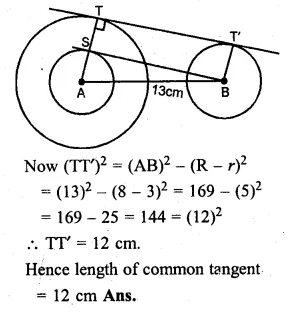Guru

# Question 15. Calculate the length of a direct common tangent to two circles of radii 3 cm and 8 cm with their centres 13 cm apart.

• 1

One of the most important and exam oriented question from Chapter name- circles
Topic – Angle properties of circles
Chapter number- 15

It is given that two circles are 13 cm apart with their centres. Radii of them are 3 cm and 8 cm.

We have to calculate the length of a direct common tangent to them.

ICSE Avichal publication

Understanding ICSE Mathematics
Question 15

Share